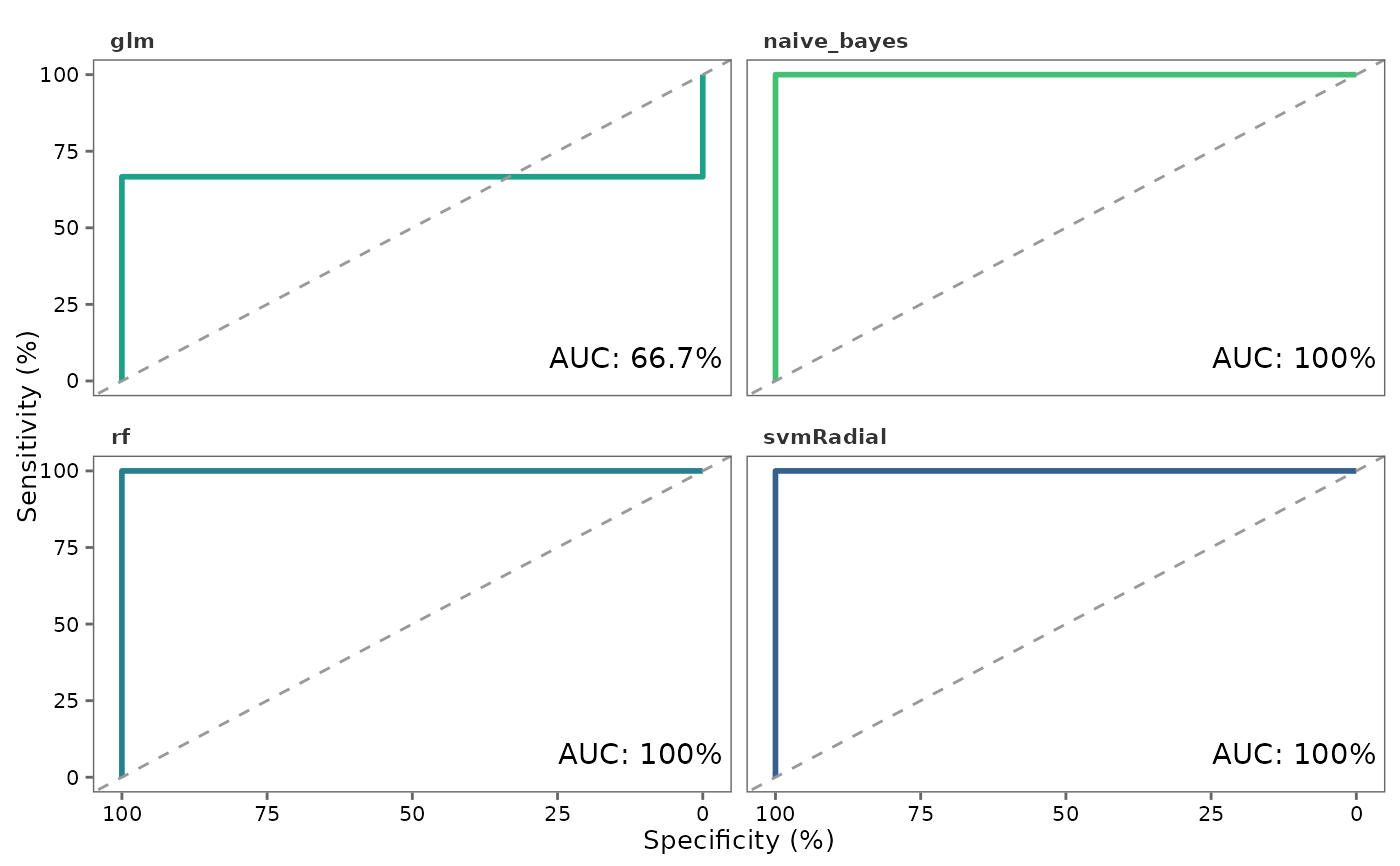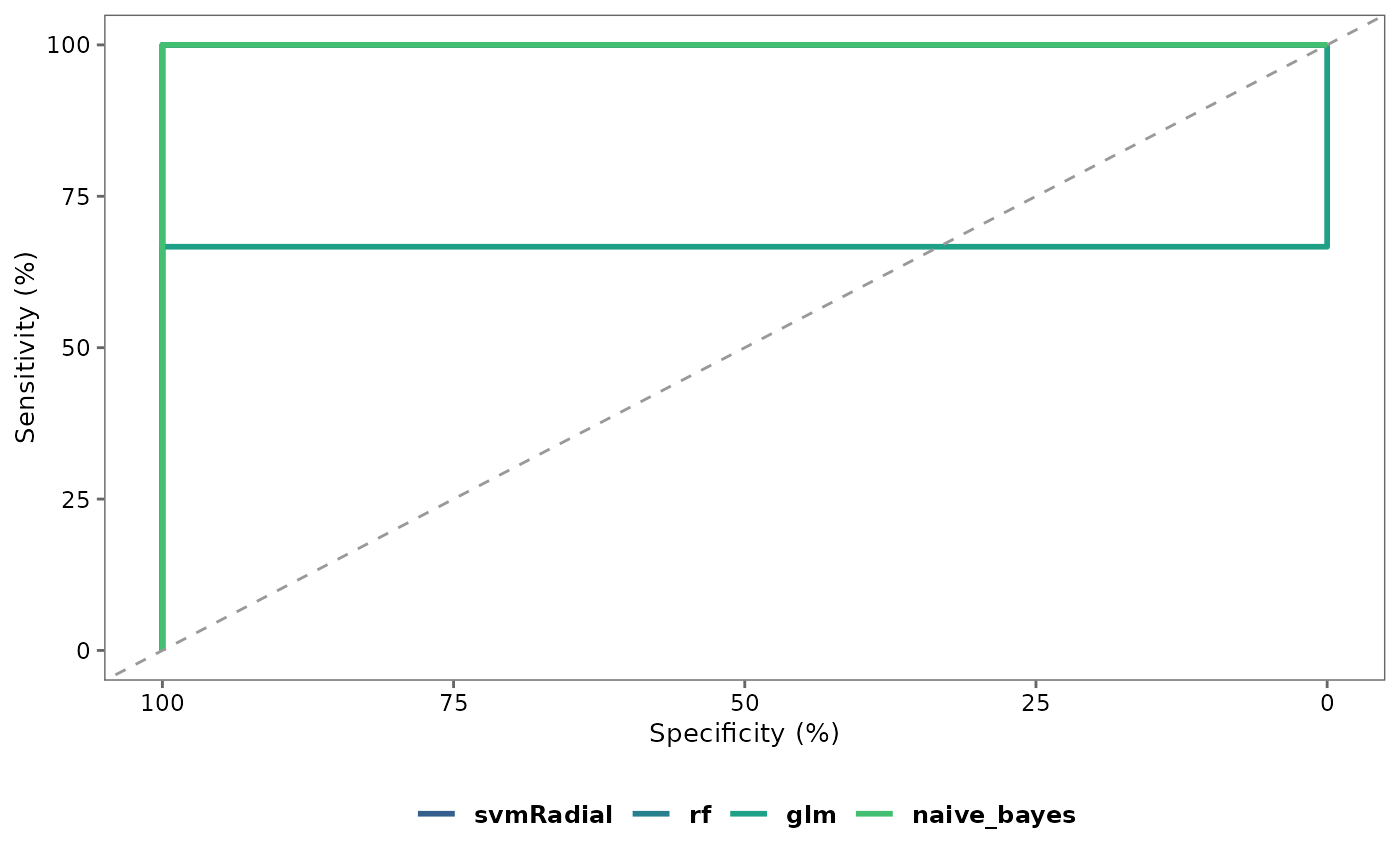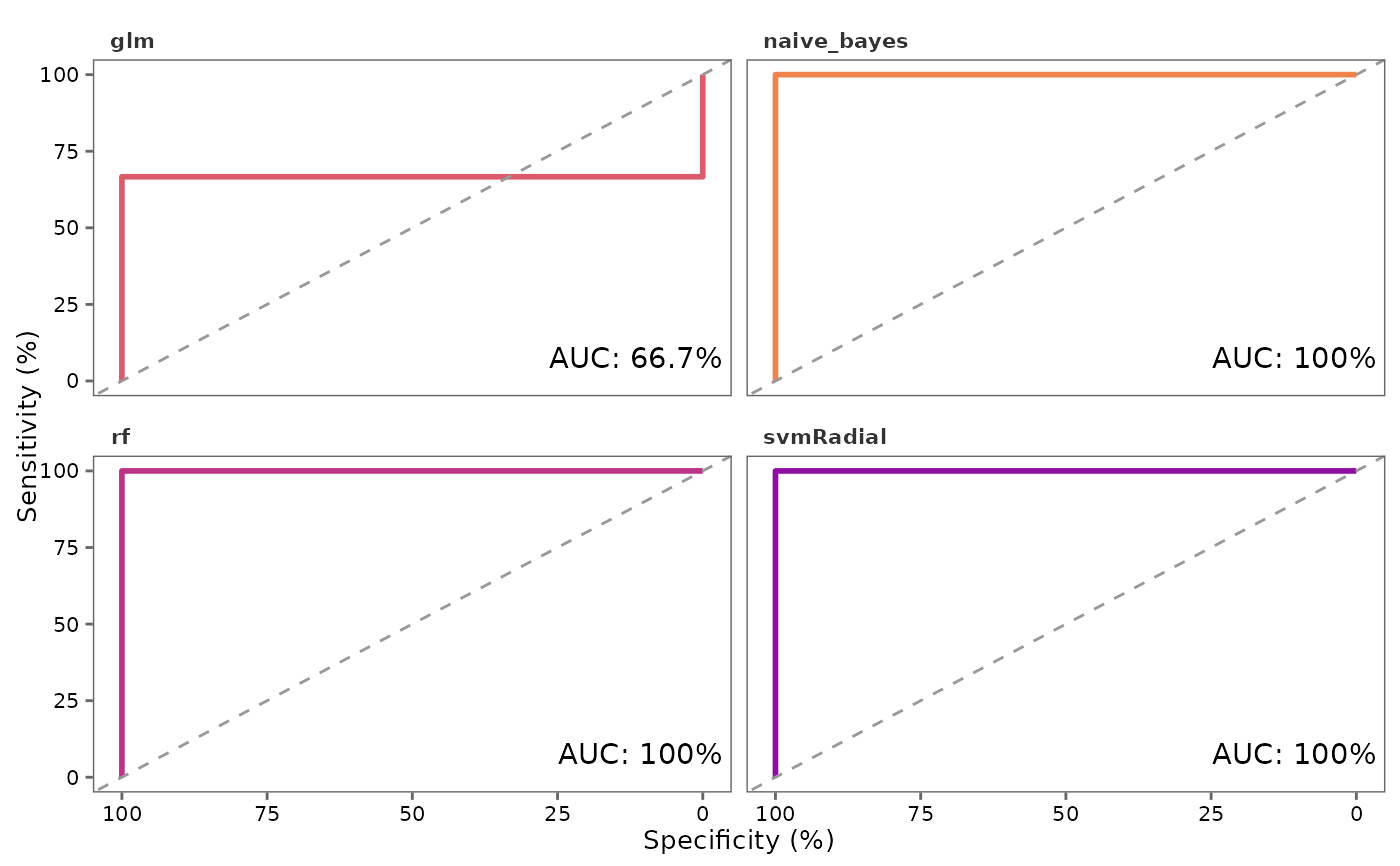This function generates Receiver Operating Characteristic (ROC) curves to evaluate models

## Usage

roc_plot(
probability_list,
split_df,
...,
multiple_plots = TRUE,
text_size = 10,
palette = "viridis",
save = FALSE,
file_path = NULL,
file_name = "ROC_plot",
file_type = "pdf",
plot_width = 7,
plot_height = 7,
dpi = 80
)

## Arguments

probability_list

A probability_list object from performing test_models with type = "prob".

split_df

A split_df object from performing split_data

...

Additional arguments to be passed on to roc.

multiple_plots

Logical. If FALSE plots all ROC curves representing algorithms included in the probability_list in a single plot.

text_size

Text size for plot labels, axis labels etc. Default is 10.

palette

Viridis color palette option for plots. Default is "viridis". See viridis for available options.

save

Logical. If TRUE saves a copy of the plot in the directory provided in file_path.

file_path

A string containing the directory path to save the file.

file_name

File name to save the plot. Default is "ROC_plot."

file_type

File type to save the plot. Default is "pdf".

plot_width

Width of the plot. Default is 7.

plot_height

Height of the plot. Default is 7.

dpi

Plot resolution. Default is 80.

## Value

A ggplot2 object.

## Details

• Next, relevant information is extracted from the ROC object to plot the ROC curves.

• test_models

• roc

## Author

Chathurani Ranathunge

## Examples

# \donttest{
## Create a model_df object
covid_model_df <- pre_process(covid_fit_df, covid_norm_df)
#> Total number of differentially expressed proteins (8) is less than n_top.
#> None of the proteins show high pair-wise correlation.
#>
#> No highly correlated proteins to be removed.

## Split the data frame into training and test data sets
covid_split_df <- split_data(covid_model_df)

## Fit models using the default list of machine learning (ML) algorithms
covid_model_list <- train_models(covid_split_df)
#>
#>
#> Running rf...
#>
#> Running glm...
#> Warning: glm.fit: algorithm did not converge
#> Warning: glm.fit: fitted probabilities numerically 0 or 1 occurred
#> Warning: glm.fit: algorithm did not converge
#> Warning: glm.fit: fitted probabilities numerically 0 or 1 occurred
#> Warning: glm.fit: algorithm did not converge
#> Warning: glm.fit: fitted probabilities numerically 0 or 1 occurred
#> Warning: glm.fit: algorithm did not converge
#> Warning: glm.fit: fitted probabilities numerically 0 or 1 occurred
#> Warning: glm.fit: algorithm did not converge
#> Warning: glm.fit: fitted probabilities numerically 0 or 1 occurred
#> Warning: glm.fit: algorithm did not converge
#> Warning: glm.fit: fitted probabilities numerically 0 or 1 occurred
#> Warning: glm.fit: algorithm did not converge
#> Warning: glm.fit: fitted probabilities numerically 0 or 1 occurred
#> Warning: glm.fit: algorithm did not converge
#> Warning: glm.fit: fitted probabilities numerically 0 or 1 occurred
#> Warning: glm.fit: algorithm did not converge
#> Warning: glm.fit: fitted probabilities numerically 0 or 1 occurred
#> Warning: glm.fit: algorithm did not converge
#> Warning: glm.fit: fitted probabilities numerically 0 or 1 occurred
#> Warning: glm.fit: algorithm did not converge
#> Warning: glm.fit: fitted probabilities numerically 0 or 1 occurred
#> Warning: glm.fit: algorithm did not converge
#> Warning: glm.fit: fitted probabilities numerically 0 or 1 occurred
#> Warning: glm.fit: algorithm did not converge
#> Warning: glm.fit: fitted probabilities numerically 0 or 1 occurred
#> Warning: glm.fit: algorithm did not converge
#> Warning: glm.fit: fitted probabilities numerically 0 or 1 occurred
#> Warning: glm.fit: algorithm did not converge
#> Warning: glm.fit: fitted probabilities numerically 0 or 1 occurred
#> Warning: glm.fit: algorithm did not converge
#> Warning: glm.fit: fitted probabilities numerically 0 or 1 occurred
#> Warning: glm.fit: algorithm did not converge
#> Warning: glm.fit: fitted probabilities numerically 0 or 1 occurred
#> Warning: glm.fit: algorithm did not converge
#> Warning: glm.fit: fitted probabilities numerically 0 or 1 occurred
#> Warning: glm.fit: algorithm did not converge
#> Warning: glm.fit: fitted probabilities numerically 0 or 1 occurred
#> Warning: glm.fit: algorithm did not converge
#> Warning: glm.fit: fitted probabilities numerically 0 or 1 occurred
#> Warning: glm.fit: algorithm did not converge
#> Warning: glm.fit: fitted probabilities numerically 0 or 1 occurred
#> Warning: glm.fit: algorithm did not converge
#> Warning: glm.fit: fitted probabilities numerically 0 or 1 occurred
#> Warning: glm.fit: algorithm did not converge
#> Warning: glm.fit: fitted probabilities numerically 0 or 1 occurred
#> Warning: glm.fit: algorithm did not converge
#> Warning: glm.fit: fitted probabilities numerically 0 or 1 occurred
#> Warning: glm.fit: algorithm did not converge
#> Warning: glm.fit: fitted probabilities numerically 0 or 1 occurred
#> Warning: glm.fit: algorithm did not converge
#> Warning: glm.fit: fitted probabilities numerically 0 or 1 occurred
#> Warning: glm.fit: algorithm did not converge
#> Warning: glm.fit: fitted probabilities numerically 0 or 1 occurred
#> Warning: glm.fit: algorithm did not converge
#> Warning: glm.fit: fitted probabilities numerically 0 or 1 occurred
#> Warning: glm.fit: algorithm did not converge
#> Warning: glm.fit: fitted probabilities numerically 0 or 1 occurred
#> Warning: glm.fit: algorithm did not converge
#> Warning: glm.fit: fitted probabilities numerically 0 or 1 occurred
#> Warning: glm.fit: algorithm did not converge
#> Warning: glm.fit: fitted probabilities numerically 0 or 1 occurred
#>
#> Running xgbLinear...
#>
#> Running naive_bayes...
#> Done!

# Test a list of models on a test data set and output class probabilities,
covid_prob_list <- test_models(covid_model_list, covid_split_df, type = "prob")
#>
#>
#> Testing rf...
#>
#> Testing glm...
#>
#> Testing xgbLinear...
#>
#> Testing naive_bayes...
#>
#> Done!

## Plot ROC curves separately for each ML algorithm
roc_plot(covid_prob_list, covid_split_df)
#> Setting levels: control = Non.Severe, case = Severe
#> Setting direction: controls > cases
#> Setting levels: control = Non.Severe, case = Severe
#> Setting direction: controls > cases
#> Setting levels: control = Non.Severe, case = Severe
#> Setting direction: controls > cases
#> Setting levels: control = Non.Severe, case = Severe
#> Setting direction: controls > cases
#> Setting levels: control = Non.Severe, case = Severe
#> Setting direction: controls > cases
#> Warning: number of columns of result is not a multiple of vector length (arg 3)
#> Warning: number of columns of result is not a multiple of vector length (arg 3)## Plot all ROC curves in one plot
roc_plot(covid_prob_list, covid_split_df, multiple_plots = FALSE)
#> Setting levels: control = Non.Severe, case = Severe
#> Setting direction: controls > cases
#> Setting levels: control = Non.Severe, case = Severe
#> Setting direction: controls > cases
#> Setting levels: control = Non.Severe, case = Severe
#> Setting direction: controls > cases
#> Setting levels: control = Non.Severe, case = Severe
#> Setting direction: controls > cases
#> Setting levels: control = Non.Severe, case = Severe
#> Setting direction: controls > cases
#> Warning: number of columns of result is not a multiple of vector length (arg 3)
#> Warning: number of columns of result is not a multiple of vector length (arg 3)## Change color palette
roc_plot(covid_prob_list, covid_split_df, palette = "plasma")
#> Setting levels: control = Non.Severe, case = Severe
#> Setting direction: controls > cases
#> Setting levels: control = Non.Severe, case = Severe
#> Setting direction: controls > cases
#> Setting levels: control = Non.Severe, case = Severe
#> Setting direction: controls > cases
#> Setting levels: control = Non.Severe, case = Severe
#> Setting direction: controls > cases
#> Setting levels: control = Non.Severe, case = Severe
#> Setting direction: controls > cases
#> Warning: number of columns of result is not a multiple of vector length (arg 3)
#> Warning: number of columns of result is not a multiple of vector length (arg 3)# }